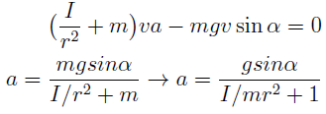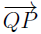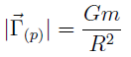# 2017PUPC普林斯顿大学物理竞赛Onsite答案免费下载

### Onsite现场竞赛部分### 2017PUPC普林斯顿大学物理竞赛完整版真题免费下载

Onsite现场竞赛部分此卷共4题

### 2017PUPC普林斯顿大学物理竞赛完整版答案免费下载

Problem 1. Warm-Up (15 points total)

a) For a general body with mass m, radius r, and moment of inertia I, the energy of the rolling body is:

E = Iw2/2 + mv2/2 + mgh  (1 point)

The bodies are rolling with out slipping, thus v =wr. Let α be the angle of the incline → h = ysinα, where y is the vertical position of the body with respect to the bottom of the incline. So:

E =1/2(I + mr2w2) + mgysinα =1/2(I + mr2)(v2/r2) + mgysinα

Energy is conserved, so dE/dt = 0: Computing the derivative and recalling that dy/dt = v and dv/dt = a we get:(3 points)

The first body to arrive at the bottom is the one with the greatest acceleration, which is equivalent to the body with the minimum I/mr2.
For the hollow pipe: I/mr2 = ma2/ma2 = 1
For the solid sphere: I/mr2 =[2/5m(2a)2]/m(2a)2 = 2/5
For the solid pipe: I/mr2 = [1/2(2m)a2]/(2m)a2 = 1/2
So the solid sphere will arrive fi rst. (1 point)
Note: this problem can also be solved using torques.

b) Let the point have mass m. This problem is easily solved using the principle of superposition. Since the gravitational force is linear in mass, the point where the mass was removed can be thought of holding two masses - the initial mass m, plus a point mass of mass -m.

The field of the 2018 evenly space point masses is 0 at P. So the only component comes from the negative mass we introduced.

Suppose the negative mass is located at point Q; thenbe the vector connecting the negative mass to point P. Therefore:(0.5 points)(0.5 points)
Four points are awarded for the explanation.

c) The points of equilibrium are given by dU/dx= 0. Therefore:(1 point)
An equilibrium is stable if U is at a local minimum (d2U/dx2 > 0) and unstable if U is at a local maximum ( d2U/dx2 < 0). Computing d2U/dx2 = 2ex cosx yields:is a stable equilibrium (1 point)

Since x∈ [0; 2π), we also need to check the point 0 to see if it is a point of local minimum or maximum of U. (1 point for identifying x = 0 as a point of equilibrium.)

[d2U/dx2 ](0) = 2e0 cos0 = 2 > 0

Therefore, x = 0 is a point of local minimum, and the equilibrium is stable. (1 point)